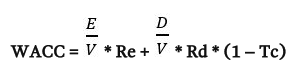Accounting

# How to Calculate Weighted Average Cost of Capital?

Weighted average cost of capital (WACC) is the average after tax cost of a company’s various capital sources, including common stock, preferred stock, bonds and any other long-term debt. A company has two primary sources of financing – debt and equity – and, in simple terms, WACC is the average cost of raising that money.

WACC is calculated by multiplying the cost of each capital source (debt and equity) by its relevant weight, and then adding the products together to determine the WACC value:Where:

• Re = cost of equity
• Rd = cost of debt
• E = market value of the firm’s equity
• D = market value of the firm’s debt
• V = E + D
• E/V = percentage of financing that is equity
• D/V = percentage of financing that is debt
• Tc = corporate tax rate

When calculating a firm’s WACC, the first step is to determine what proportion of a firm is financed by equity and what proportion is financed by debt by entering the appropriate values into the (E/V )and (D/V) components of the equation. Next, the proportion of equity (E/V) is multiplied by the cost of equity (Re); and the proportion of debt (D/V) is multiplied by the cost of debt (Rd).

The debt side of the equation (D/V* Rd) is then multiplied by (1 – Tc) to get the after-tax cost of debt (there is a tax shield associated with interest). The final step is to add the equity side of the equation to the debt side of the equation to determine WACC.

For example, a firm’s financial data shows the following:

• Equity = \$8,000
• Debt = \$2,000
• Re = 12.5%
• Rd = 6%
• Tax rate = 30%

To find WACC, enter the values into the equation and solve:Because the calculation takes time, most investors use online stock analysis tools to find a company’s WACC.